# AP Physics C: Mechanics : Using Pendulum Equations

## Example Questions

### Example Question #1 : Using Pendulum Equations

A simple pendulum of lengthswings at a radiusfrom a fixed point on the ceiling. As it moves, the pendulum creates an angle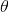with the line that extends vertically downwards from the fixed point. At what angle does the mass attached to the pendulum have the highest kinetic energy?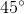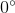Explanation:

The correct answer is. Since the pendulum is at the bottom of its motion at this point, it has the lowest amount of energy given to gravitational potential and thus, the highest kinetic energy.

### Example Question #45 : Ap Physics C

If a simple pendulum is constructed using a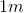rope of negligible weight and a large steel ball weighing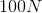. What is the period of the pendulum?

Not enough information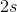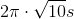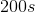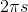Explanation:

Use the equation for the period of a simple pendulum: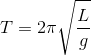Here,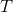is the period in seconds,is the length of the pendulum in meters, and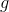is the acceleration due to gravity in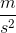.

We can plug in the given quantities for length and acceleration to solve for the period.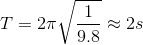Note that the mass of the rope as negligible. We also need not incorporate the extraneous information regarding the weight of the steel ball attached to the pendulum. This does not influence the period of this simple pendulum.